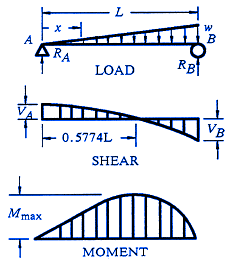Beam Deflection, Stress, Strain Equations and Calculators

Beam Stress Deflection Equations / Calculator with Pinned End and Simply Supported End Tapering LoadingALL calculators require a Premium Membership

Reaction and Shear EquationBending Moments EquationDeflection and End Slope EquationWhere:

 E = Modulus of Elasticity psi (N/mm2) I = Moment of Inertia in4 (mm4) W = Load, Total lbf (N) w = Unit Load lbs/in (N/mm) y = Deflection inches (mm) a, b, c, d, x, L = Some distance as indicated inches (mm) n = Distance neutral axis inches (mm) Vmax = Shear Load lbf (N) Mmax = Moment lbs-in (N-mm) θmax = Slope Angle radian (radian) σmax = Stress max. psi (N/mm2)
• Deflections apply only to constant cross sections along entire length.

References:

• Boeing Design Manual, Rev G. 1994 BDMMembership Register | LoginHomeEngineering Book StoreEngineering ForumExcel App. DownloadsOnline Books & ManualsEngineering NewsEngineering VideosEngineering CalculatorsEngineering ToolboxGD&T Training Geometric Dimensioning TolerancingDFM DFA TrainingTraining Online EngineeringAdvertising CenterCopyright Notice